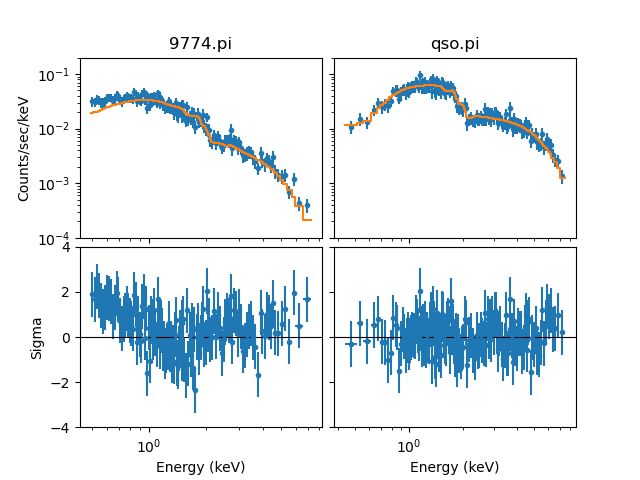# How can I create multiple plots in the same window?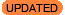New in CIAO 4.13

New in CIAO 4.13 is the ability to set options for all plots in the plot call. The display of residual axes are now always drawn with a linear scale, even if set_ylog has been used.

In the following we assume you have two data sets loaded with ids of 1 (i.e. the default) and 2, that you have done the following

```sherpa> set_xlog()
sherpa> set_ylog()
```

and that fits have been made to both data sets.

If you wish to display multiple datasets in the same plot window then you can use the plot command; for example if you have two datasets with ids of 1 and 2 then:

```sherpa> plot("data", 1, "data", 2)
```

will display each data set in a separate plot in the same window (Figure 1).

### Plotting multiple data sets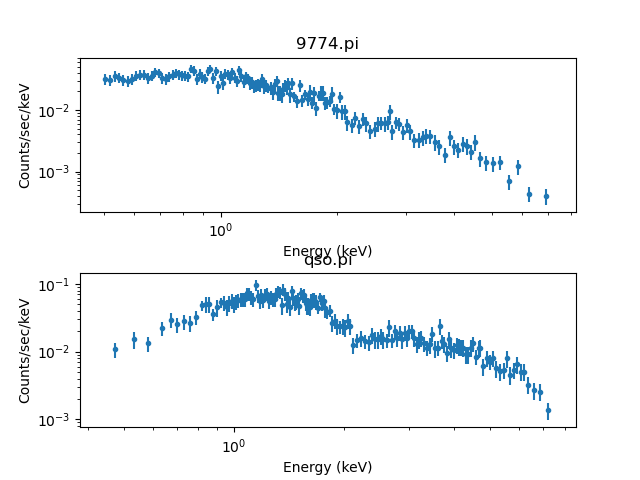### Plotting multiple data sets

The default layout of the plots is not guaranteed to be free of overlaps of plot elements, such as the X-axis label of the top plot and the title of the second plot.

As of CIAO 4.13 we can now also adjust all the plots, such as over-riding the set_xlog with:

```sherpa> plot("data", 1, "data", 2, xlog=False)
```

After such a call, matplotlib functions can be used to tweak the layout, sich as increasing the vertical space between the two plots (Figure 2):

```sherpa> plt.subplots_adjust(hspace=0.5)
```

### Increasing the vertical space between the plots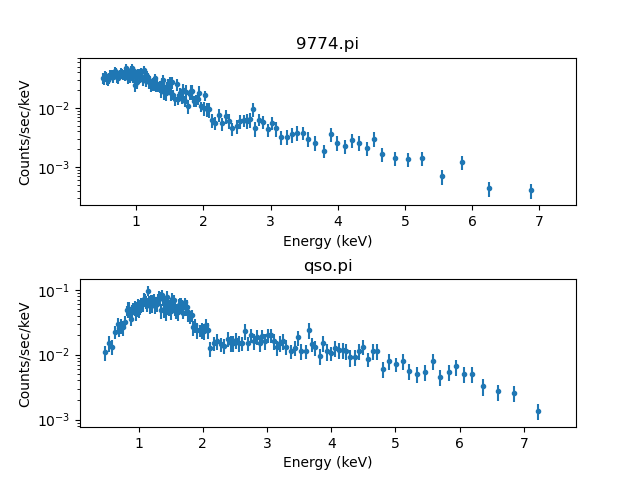### Increasing the vertical space between the plots

You can display more than two plots, for instance (Figure 3):

```sherpa> plot('fit', 1, 'fit', 2, 'delchi', 1, 'delchi', 2)
```

### Plotting fit and residuals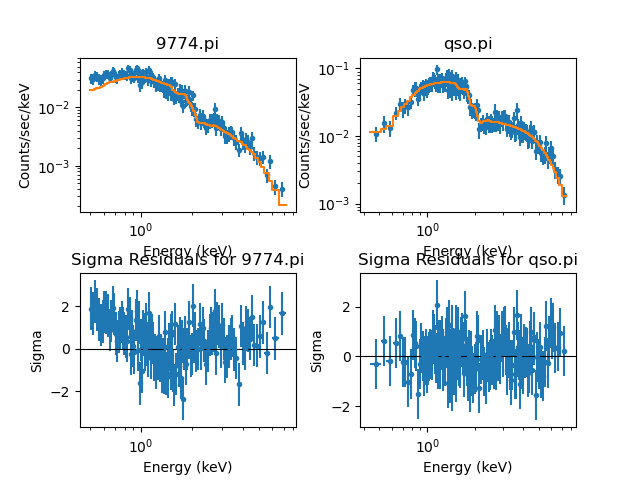### Plotting fit and residuals

The overlapping of labels is present and the Y axes of the plots are also not the same, which makes cross comparison difficult.

As with the previous example, matplotlib commands can be used to tweak the appearance of the plot (Figure 4):

```sherpa> fig = plt.gcf()
sherpa> for i in [0, 1]:
...:    fig.axes[i].xaxis.label.set_visible(False)
...:    fig.axes[i].xaxis.set_ticklabels([])
...:    fig.axes[i].set_ylim(1e-4, 2e-1)
...:
sherpa> for i in [2, 3]:
...:    fig.axes[i].title.set_visible(False)
...:    fig.axes[i].set_ylim(-4, 4)
...:
sherpa> for i in [1, 3]:
...:    fig.axes[i].yaxis.label.set_visible(False)
...:    fig.axes[i].yaxis.set_ticklabels([])
...: